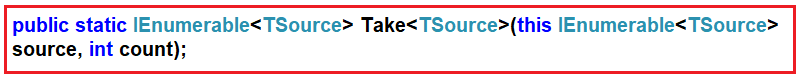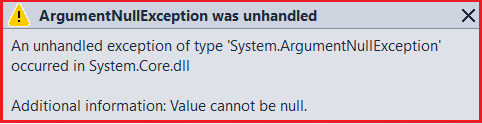# Take Operator in Linq

## Take Operator in Linq with Examples

In this article, I am going to discuss the Take Operator in Linq with some examples. Please read our previous article before proceeding to this article where we gave a brief introduction to Partitioning Operators in Linq. As part of this article, we are going to discuss the following concepts related to the Take Operator in C#.

1. What is Take Operator and its need in C#.NET?
2. Examples using Take Operator.
3. Take Operator in LINQ using Filter.
4. What happens when Applying the Where Operator after the Take Operator?
5. Take Method on the Data Source which is null?

#### What is Take Operator and its need in C#.NET?

The Take Operator in Linq is used to fetch the first “n” number of elements from the data source where “n” is an integer which is passed as a parameter to the Take method. There is only one version available for this Take method as shown below.As you can see in the above image, the take method takes one integer count parameter and this method going to return that number of contiguous elements from the data source. If the data source is null then it is going to throw an exception.

You can use the Take method with both the Method and Query Syntax but the most important point that you need to remember is it will not make any changes to the positions of the elements.

##### Take Method in Linq with Method Syntax:

In the following example, we created one integer collection which contains 10 elements. Then we retrieve the first 4 elements from the collection using the Method syntax.

```using System;
using System.Collections.Generic;
using System.Linq;

namespace LINQDemo
{
class Program
{
static void Main(string[] args)
{
List<int> numbers = new List<int>() {1, 2, 3, 4, 5, 6, 7, 8, 9, 10 };
List<int> ResultMS = numbers.Take(4).ToList();

foreach(var num in ResultMS)
{
Console.Write(\$"{num} ");
}

}
}
}
```

Output: 1 2 3 4

##### Take Method in Linq with Query Syntax:

Let us rewrite the previous example using query syntax. There is no such operator called Take is available to write the query syntax, So, here we need to use the mixed syntax as shown below.

```using System;
using System.Collections.Generic;
using System.Linq;

namespace LINQDemo
{
class Program
{
static void Main(string[] args)
{
List<int> numbers = new List<int>() {1, 2, 3, 4, 5, 6, 7, 8, 9, 10 };
List<int> ResultQS = (from num in numbers
select num).Take(4).ToList();

foreach(var num in ResultQS)
{
Console.Write(\$"{num} ");
}

}
}
}
```

Output: 1 2 3 4

##### Take Method with Filtering Operator in C#.NET:

Our requirement is to take four elements from the collection but the condition is that the elements should be greater than 3. In such cases, first, we need to filter the data using Where operator and then we need to take the data using Take operator as shown in the below example.

```using System;
using System.Collections.Generic;
using System.Linq;

namespace LINQDemo
{
class Program
{
static void Main(string[] args)
{
List<int> numbers = new List<int>() {1, 2, 3, 4, 5, 6, 7, 8, 9, 10 };

//Using Method Syntax
int[] ResultMS = numbers.Where(num => num > 3).Take(4).ToArray();

//Using Mixed Syntax
List<int> ResultQS = (from num in numbers
where num > 3
select num).Take(4).ToList();

foreach(var num in ResultMS)
{
Console.Write(\$"{num} ");
}

}
}
}```

Output: 4 5 6 7

##### Applying Filtering after the Take method in C#:

If you apply the Filtering Operator after the Take method then you will not get the result as expected as shown in the below example.

```using System;
using System.Collections.Generic;
using System.Linq;

namespace LINQDemo
{
class Program
{
static void Main(string[] args)
{
List<int> numbers = new List<int>() {1, 2, 3, 4, 5, 6, 7, 8, 9, 10 };

//Using Method Syntax
int[] ResultMS = numbers.Take(4).Where(num => num > 2).ToArray();

//Using Mixed Syntax
List<int> ResultQS = (from num in numbers
select num).Take(4).Where(num => num > 2).ToList();

foreach(var num in ResultQS)
{
Console.Write(\$"{num} ");
}

}
}
}
```

Output: 3 4

As you can see in the above output, we are getting only 2 elements. This is because of the wrong use of Where operator. In this case, first, the “Take” method is executed which will return four elements and then on this result set the “Where” method is applied which further filter the data based on the condition specified. So, it is always a good programming practice to use the Where operator first and then the Take operator.

##### What happens when the Data Source is null?

When we are applying the Take operator on a data source which is null, then we will get an exception i.e. ArgumentNullException as shown in the below example.

```using System;
using System.Collections.Generic;
using System.Linq;

namespace LINQDemo
{
class Program
{
static void Main(string[] args)
{
//data source is null
List<int> numbers = null;

int[] ResultMS = numbers.Where(num => num > 3).Take(4).ToArray();

foreach(var num in ResultMS)
{
Console.Write(\$"{num} ");
}